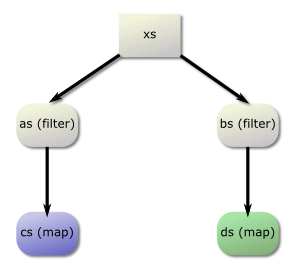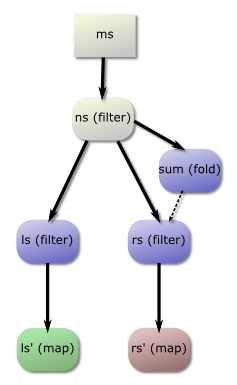# Errata

In the paper Fusing filters with integer linear programming, we convert combinator programs to linear programs to find the best fusion clustering. It may seem reasonable to only allow fusion between combinators of the same size, but the problem is filters have an unknown output size.

Instead, we restrict fusion so that two combinators of different sizes may only be fused together if they have some ancestor nodes of the same size, and all four are fused together. We define a function `parents` to classify these ancestor nodes of the same size, but the paper definition of `parents` is incorrect and in some cases returns multiple ancestors. If there are multiple ancestor nodes of the same size, choosing the wrong ones may unnecessarily prohibit fusion.

Below, I will show an example of how `parents` is used, an example where the paper definition can give poor fusion, and finally a fixed definition of `parents`.

## Simple example

Let us first look at how a simple function is fused.

``````fun xs
= let as  = filter (>0) xs
bs  = filter (<0) xs
cs  = map    (+1) as
ds  = map    (-1) bs
in (cs, ds)``````Graph of `fun`

Notice that `cs` and `ds` have different loop sizes, and so are coloured differently in the graph. Both `as` and `bs` have the same size and can be fused together; `as` can be fused into `cs` and `bs` can be fused into `ds`. However, `cs` and `ds` can only be fused together if `as` and `bs` are in the same group.

In this case, `parents cs ds = [(as,bs)]`, meaning that `cs` and `ds` may only be fused together if `as`, `bs`, `cs` and `ds` are all fused together.

## Multiple parents

In the case of a vertical line of filters, a pair of nodes may have multiple ancestors with the same size. Choosing the correct ancestors is particularly important when there are fusion-preventing edges.

``````filts ms
= let ns  = filter (>0)    ms

sum = fold   (+) 0   ns
ls  = filter (>sum)  ns
rs  = filter odd     ns

ls' = map    (+1)    ls
rs' = map    (-1)    rs
in  (ls', rs')``````Graph of `filts`

Here, the question is whether `ls'` and `rs'` can be fused together. There are multiple ancestors with the same type: `(ls,rs)` and `(ns,ns)`. Choosing `(ns,ns)` will actually disallow `ls'` and `rs'` from being fused together, because of the fusion-preventing path between `ns` and `rs'`. On the other hand, choosing `(ls,rs)` allows fusion. In this case, `(ls,rs)` is the correct choice.

## Solution

One simple solution is to modify `parents` to choose the “closest” ancestors when there are multiple. Given the original definition:

``````parents a b
| size a == size b
=  [ (a, b) ]
| otherwise
=  [ parents a' b  | a' <- trans a ]
++ [ parents a  b' | b' <- trans b ]``````

This can be modified to keep track of distance, and return the minimum ancestor:

``````parents a b
= map fst
\$ parents' a b 0

parents' a b dist
| size a == size b
=  [ ((a, b), dist) ]

| otherwise
= sortBy (compare `on` snd)
\$  [ parents a' b  (dist+1) | a' <- trans a ]
++ [ parents a  b' (dist+1) | b' <- trans b ]``````
October 12, 2014